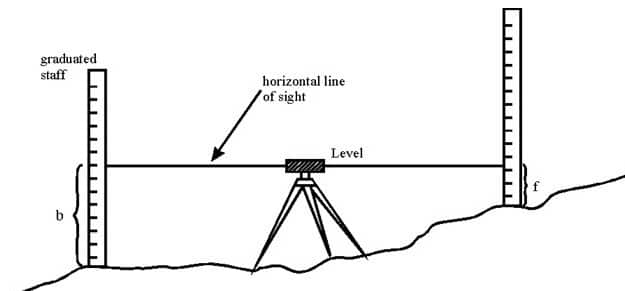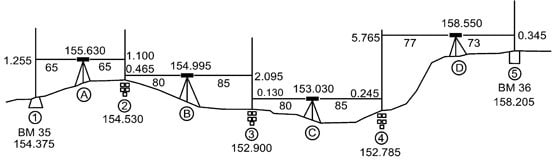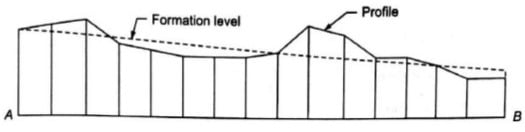# Levelling Survey

Leveling is a branch of surveying in civil engineering to measure levels of different points with respect to a fixed point such as elevation of a building, height of one point from ground etc.

### Direct Leveling

It is the most commonly used method of leveling. In this method, measurements are observed directly from leveling instrument.

Based on the observation points and instrument positions direct leveling is divided into different types as follows:

• Simple leveling
• Differential leveling
• Fly leveling
• Profile leveling
• Precise leveling
• Reciprocal leveling

#### Simple Leveling

It is a simple and basic form of leveling in which the leveling instrument is placed between the points which elevation is to be find. Leveling rods are placed at that points and sighted them through leveling instrument. It is performed only when the points are nearer to each other without any obstacles.#### Differential Leveling

Differential leveling is performed when the distance between two points is more. In this process, number of inter stations are located and instrument is shifted to each station and observed the elevation of inter station points. Finally difference between original two points is determined.#### Fly Leveling

Fly leveling is conducted when the benchmark is very far from the work station. In such case, a temporary bench mark is located at the work station which is located based on the original benchmark. Even it is not highly precise it is used for determining approximate level.#### Profile Leveling

Profile leveling is generally adopted to find elevation of points along a line such as for road, rails or rivers etc. In this case, readings of intermediate stations are taken and reduced level of each station is found. From this cross section of the alignment is drawn.#### Precise Leveling

Precise leveling is similar to differential leveling but in this case higher precise is wanted. To achieve high precise, serious observation procedure is performed. The accuracy of 1 mm per 1 km is achieved.

#### Reciprocal Leveling

When it is not possible to locate the leveling instrument in between the inter visible points, reciprocal leveling is performed. This case appears in case of ponds or rivers etc. in case of reciprocal leveling, instrument is set nearer to 1st station and sighted towards 2nd station.### Trigonometric Leveling

The process of leveling in which the elevation of point or the difference between points is measured from the observed horizontal distances and vertical angles in the field is called trigonometric leveling.In this method, trigonometric relations are used to find the elevation of a point from angle and horizontal distance so, it is called as trigonometric leveling. It is also called as indirect leveling.

### Barometric Leveling

Barometer is an instrument used to measure atmosphere at any altitude. So, in this method of leveling, atmospheric pressure at two different points is observed, based on which the vertical difference between two points is determined. It is a rough estimation and used rarely.